Disk diameter 500 mm & thickness 10 mm
 Comparison  with abaqus 6.4 in some example in plasticity problems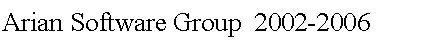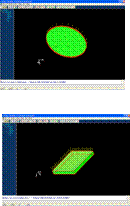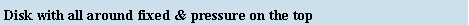Cube dimension 1000×1000 mm & thickness 100 mm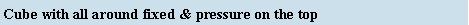Beam dimension with: 50× 10×10 mm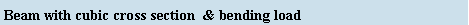Beam dimension with : 50× 10×10 mm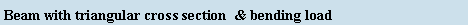Justification new method in plasticity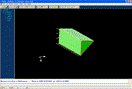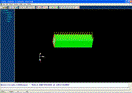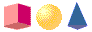E=200 Gpa  ,ν =0.3  , σ = 200×106
 E=200 Gpa  ,ν =0.3  , σ = 200×106
 E=200 Gpa  ,ν =0.3  , σ = 630×106 ε 0.25
 E=200 Gpa  ,ν =0.3  , σ = 630×106 ε 0.25